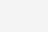# IF函数设计含发票和不含发票的计算思路1.=IF(O3=””R2-Q3,IF(O3=”不含发票”,R2-Q3,IF(O3=”含发票”,R2+L3-Q3)))

2.=IF(OR(O3=””,O3=”不含发票”),R2-Q3,R2+L3-Q3)

3.=IF(O3=”含发票”,R2+L3-Q3,R2-Q3)

(0)

3.9K

3.5K

9.8K

2.9K

10.8K